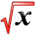# Compound Interest RateThis article is part of the MathHelp Tutoring WikiThis article is part of the EconHelp Tutoring Wiki

## General Formula

Given an annual interest rate r, we can use the number following formula to compute the increase in the principle when the interest is compound annually.

$A(t)=P(1+r)^{t}$If the interest is compounded in n times a year (in our example n=12), the amount after t years is,

$A(t)=P({\frac {1+r}{n}})^{tn}$If we take a limit as n goes to infinity, we say that interest is compounded continuously.

As we know, $e=\lim _{n\to \infty }(1+{\frac {1}{n}})^{n}$Let's use the substitution m=n/r, so n=mr

{\begin{aligned}A(t)&=\lim _{m\to \infty }P(1+{\frac {1}{m}})^{tmr}\\&=P(\lim _{m\to \infty }(1+{\frac {1}{m}})^{tmr}\\&=P(\lim _{m\to \infty }((1+{\frac {1}{m}})^{m})^{tr}\\&=Pe^{tr}\end{aligned}}## Practice Question

Q: say P=\$10,000 with r=0.06 and t=3, how much will you have if interest is compounded.

(1) 6 times a year

(2) 24 times a year

(3) continuously

A:

(1) $A=P(1+r/n)^{tn}=10000(1+0.06/6)^{3\times 6}=11961.47$(2) $A=10000(1+0.06/24)^{3\times 24}=11969.48$(3) $A=Pe^{rt}=10000e^{0.06\times 3}=11972.17$